[BACK]Computer Modeling in Engineering & SciencesDOI: 10.32604/cmes.2022.017532

ARTICLE

Parameter Estimation Based on Censored Data under Partially Accelerated Life Testing for Hybrid Systems due to Unknown Failure Causes

Department of Basic Sciences, College of Science and Theoretical Studies, Saudi Electronic University, Dammam, 32256, Saudi Arabia
*Corresponding Author: Mustafa Kamal. Email: m.kamal@seu.edu.sa; kamal19252003@gmail.com
Received: 18 May 2021; Accepted: 11 October 2021

Abstract: In general, simple subsystems like series or parallel are integrated to produce a complex hybrid system. The reliability of a system is determined by the reliability of its constituent components. It is often extremely difficult or impossible to get specific information about the component that caused the system to fail. Unknown failure causes are instances in which the actual cause of system failure is unknown. On the other side, thanks to current advanced technology based on computers, automation, and simulation, products have become incredibly dependable and trustworthy, and as a result, obtaining failure data for testing such exceptionally reliable items have become a very costly and time-consuming procedure. Therefore, because of its capacity to produce rapid and adequate failure data in a short period of time, accelerated life testing (ALT) is the most utilized approach in the field of product reliability and life testing. Based on progressively hybrid censored (PrHC) data from a three-component parallel series hybrid system that failed to owe to unknown causes, this paper investigates a challenging problem of parameter estimation and reliability assessment under a step stress partially accelerated life-test (SSPALT). Failures of components are considered to follow a power linear hazard rate (PLHR), which can be used when the failure rate displays linear, decreasing, increasing or bathtub failure patterns. The Tempered random variable (TRV) model is considered to reflect the effect of the high stress level used to induce early failure data. The maximum likelihood estimation (MLE) approach is used to estimate the parameters of the PLHR distribution and the acceleration factor. A variance covariance matrix (VCM) is then obtained to construct the approximate confidence intervals (ACIs). In addition, studentized bootstrap confidence intervals (ST-B CIs) are also constructed and compared with ACIs in terms of their respective interval lengths (ILs). Moreover, a simulation study is conducted to demonstrate the performance of the estimation procedures and the methodology discussed in this paper. Finally, real failure data from the air conditioning systems of an airplane is used to illustrate further the performance of the suggested estimation technique.

Keywords: Step stress partially accelerated life test; progressive hybrid censoring; data masking; power linear hazard rate distribution; hybrid system

1  Introduction

Computers, cellphones, and many other systems of competitive innovation and modernization are getting increasingly complex, with numerous subsystems and sub-assemblies in each. Furthermore, these subsystems and subassemblies are made up of several components, making the life testing procedure for such systems more complicated. The study of reliability and life testing of systems, subsystems, or components is entirely depend on lifetime data, which is a combination of two important pieces of information. The first is to determine the product’s failure time, and the second is to determine the reasons for its failure. The failure times of the system can easily be recorded, but the cause of the failure is not always recognized due to a variety of factors, including a lack of adequate diagnosis, time and expense constraints for comprehensive failure analysis, and numerous component failures with destructive consequences. As a result, in the literature of reliability and life testing analysis, such data in which the true cause of system failure is unknown and only a minimum random subset of the reasons that are responsible for system failure can be recognized is referred to as masked data. See [1,2] for more details.

However, there are situations when these sorts of relationships are not possible. Because of the prevalence of this issue in ALTs, PALTs are considered to be preferable option and are usually implemented in two ways. The first is constant stress PALT, while the second is SSPALT. In SSPALT, a sample of components or systems is first tested under normal usage circumstances for a certain amount of time, and then systems or components that have not failed are allocated to testing at accelerated conditions until all items fail or a predetermined censorship scheme is met. SSPALT analysis has been considered by many authors since it was first suggested by [11,12] as a TRV model. Bhattacharyya et al.  developed a tampered failure rate model using the TRV model. Bai et al.  are others who considered SSPALT using the same concept of the TRV model for different distributions and censoring schemes. Assuming the TRV model, Zhang et al.  discussed the MLEs of the unknown parameters for the extended Weibull distribution. Ismail et al. [18,19] investigated the MLEs of the parameters of the Weibull and Burr Type-XII distributions, respectively, and compared the results based on two different PrHC schemes. Mahmoud et al.  deals with SSPALT based on an adaptive type PrHC scheme to obtain estimates of parameters using MLEs and Bayesian estimates (BEs) of generalized Pareto, two-parameter exponentiated Weibull and Lindley distributions, respectively.

A considerable number of studies have been carried out on parameter estimation using mask data based on ORTs since it was first introduced by [1,2]. Considering different failure distributions, Guess et al.  utilizes the MLE technique for estimating model parameters for a single component or a series or parallel systems of two or three components, whereas Reiser et al.  considered BE technique based on different priors. As it was discussed earlier, many real-life systems or machines these days are made of hybrid structures which are a combination of series and parallel subsystems. More complex systems can have many hybrid subsystems which are generally connected in parallel-series, series-parallel, series-parallel-series, and parallel-series-parallel configurations and so on. Peng et al.  developed a Bayesian approach for system reliability evaluation and prediction in which pass-fail data, lifespan data, and deterioration data are integrated coherently at multiple system levels. Yang et al.  developed an Adaptive Bayesian Melding reliability evaluation technique for analyzing and assessing the reliability of a hierarchical system with imperfect prior knowledge. They also expanded the concept to a broader multi-level hierarchical structure by employing a more effective method of pooling inconsistent priors. Yang et al.  proposed a Bayesian reliability approach for complex systems with dependent life metrics and developed a likelihood decomposition method to convert the overall likelihood into a product of explicit and implicit evidence-based likelihood functions. As of now, only a limited number of research papers considering hybrid systems based on ORTs have been published. Considering three component parallel-series and series-parallel systems, Wang et al.  obtained the MLEs of the parameters based on masked data assuming constant and linear hazard rate of independent components. Sha et al.  considered a hybrid system of three dependent components and obtained the MLEs based on mask data assuming a bivariate exponential distribution. Cai et al.  considered the same system as in  and derived the MLEs based copula function under mask cause of failure. Recently, Rodrigues et al. [50,51] used more complex structures based on four or five components and obtained MLEs and BEs under incomplete data.

Only a few studies on ALTs so far that focused on hybrid systems and masked data have been published in available research. Reference  under SSALT, describes the procedure of obtaining MLEs of the parameters of the Weibull distribution for a series system based on an expectation minimization algorithm assuming symmetric masking. Considering masked interval data in SSALT, Fan et al.  obtained estimates of the parameters of the exponential distribution. Xu et al. [54,55] described the general Bayesian analysis of the series system masked failure data for the log-location-scale and Weibull distributions respectively under SSALT. Assuming the same exponential hazard rate for a four components hybrid system, Shi et al. [56,57] obtained the MLEs of unknown parameters of the model based on the masked data under SSPALT and CSPALT respectively. Shi et al.  considered two different hybrid systems of three components and then obtained MLEs of the modified Weibull distribution.

To the best of our knowledge so far, no article has been published that considers SSALT for PLHR distribution for a hybrid system under masked data. Much of the sources listed above considered hazard rates that are monotonic in nature, but there are cases where the hazard rate is not monotonic. The primary goal of this work is to describe the SSPALT using a more flexible PLHR that can be used when failure rates indicate non-monotonic characteristics for hybrid systems. To illustrate the considered estimation procedure under SSPALT using PrHC masked data, a three-component hybrid system is considered. The rest of the paper is organized as follows, Section 2 addresses the formulation of the SSPALT model for PrHC masked data from a hybrid system and some useful assumptions. The MLEs and corresponding ACIs and ST-B CIs of the parameters and acceleration factor are discussed in Section 3. In Section 4, we conducted a simulation study to demonstrate the performance of the estimation procedures and the methodology discussed in this paper. Section 5 addresses the suggested approach’s real-life data applicability. Finally, we conclude our study in Section 6.

2  Design and Assumptions of the Model

2.1 PLHR Distribution

In reliability and life testing experiments/studies, hazard rate is the most important function since it plays a very important role in characterizing the aging process of the systems and hence in classifying failure time distributions, more details can be seen in . Commonly used hazard rates are constant, linear and power hazard rates. However, distributions derived from these hazard rates are very useful and popular in reliability and life testing theory when the failure rate depicts monotonic properties, but they cannot be used to fit non-monotonic failure rates. Therefore, in this paper, a more flexible PLHR distribution introduced by  that is derived from the combination of linear and power hazard rates is considered. Different shapes of hazard rate and density function of PLHR distribution with different values of parameters are given in Figs. 1 and 2, respectively. The Probability density function (PDF), cumulative distribution function (CDF), reliability function (RF) and the hazard rate (HR) of PLHR distribution are given respectively by the following equations:

f(t,γ,κ)=(t+γtκ)exp(12t2γκ+1tκ+1);t>0,γ>0,κ>1&κ1 (1)

F(t)=1exp(12t2γκ+1tκ+1) (2)

R(t)=exp(12t2γκ+1tκ+1) (3)

h(t)=(t+γtκ) (4)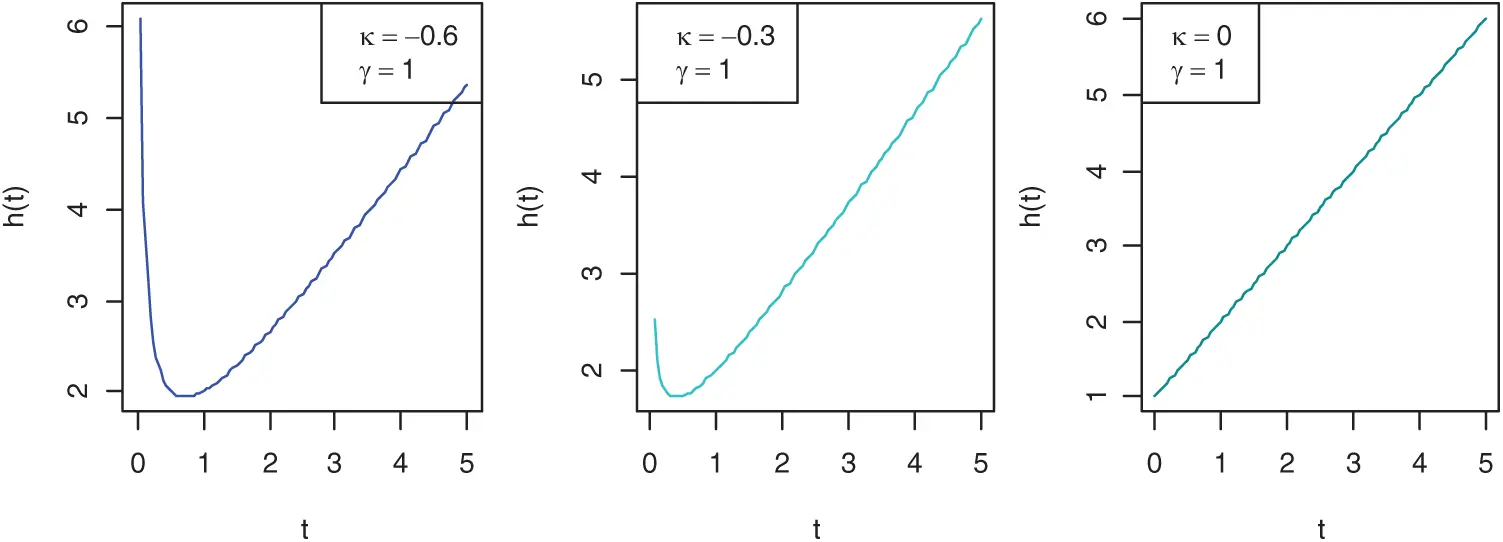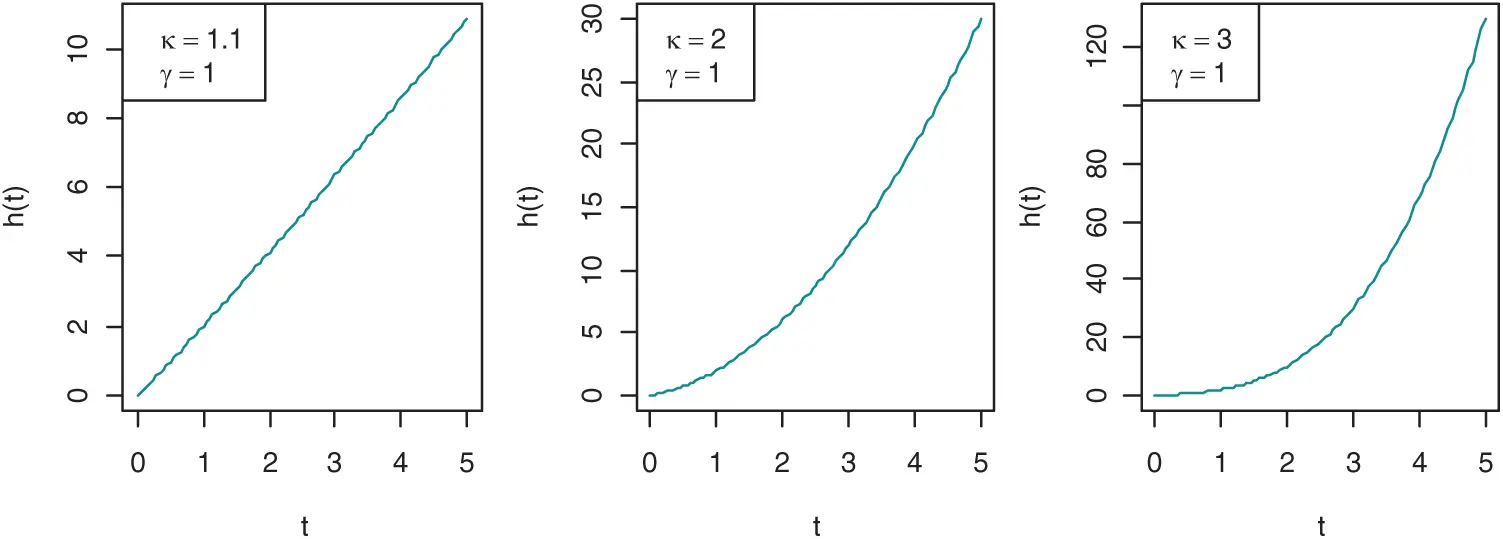Figure 1: Shapes of the HR function of PLHR distribution with different values of the parameters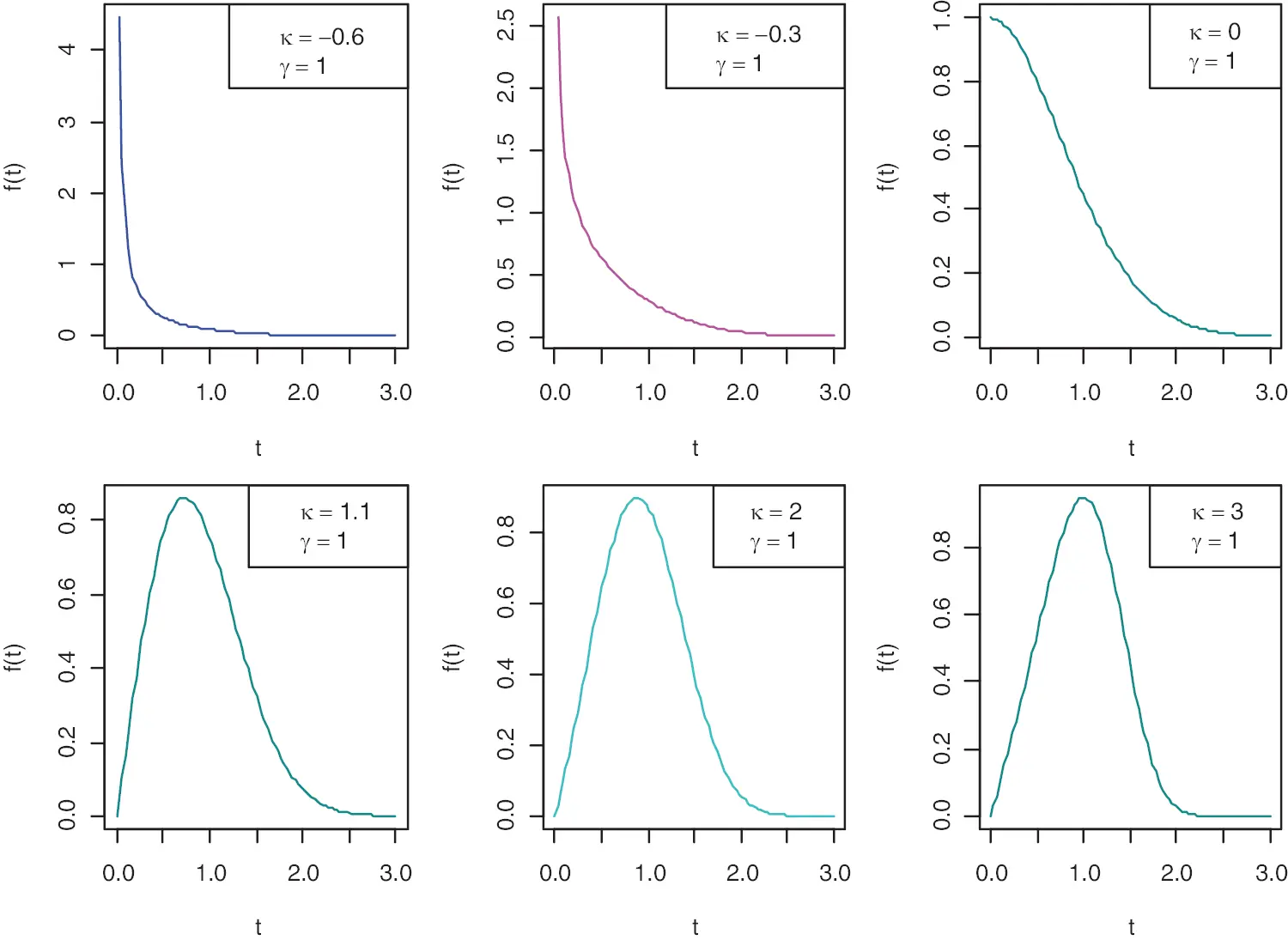Figure 2: Shapes of the PDF of PLHR distribution using different values of the parameters

2.2 Basic Assumptions

In this paper, the following assumptions are made in order to describe the SSPALT model for a hybrid structure under PrHC masked data:

1.    Xξ,ξ=1,2 represents the two stress levels, i.e., X1 and X2 are normal and accelerated stress level, respectively, used in SSPALT.

2.    The system under consideration is a series parallel system containing three independent components j = 1, 2, 3 which is described by Fig. 3 as follows:Figure 3: A series parallel hybrid system of three components

3.   The lifetime Tξi,ξ=1,2;i=1,2,,n of system i tested under SSPALT are i.i.d. at both normal and accelerated test conditions.

4.   The data masking mechanism is statistically independent of the various stress conditions used in the experiment and the actual cause of failure of the system.

5.   The lifetime of jth component at normal stress X1, in the considered hybrid system follows PLHR distribution and the corresponding PDF, CDF, SF and HR are given respectively by Eqs. (1)(4).

6.   Let the total lifetime T of a component in hybrid system under SSPALT is explained by TRV model and can be written as follows:

T={X,if X<ττ+ϑ1(Xτ),if X>τ (5)

where X represents lifetime of the component at X1, τ is the time when items are switched from X1 to X2 and is known as stress change time and ϑ>1 is used to reflect the effect of stress change and is known as acceleration factor. Now lifetime of the component at X2 by using Eqs. (1)(5) can be obtained as

f2(t,γ,κ,ϑ)=ϑ{(τ+ϑ(tτ))+γ(τ+ϑ(tτ))κ}×exp{12(τ+ϑ(tτ))2γκ+1(τ+ϑ(tτ))κ+1} (6)

F2(t,γ,κ,ϑ)=1exp{12(τ+ϑ(tτ))2γκ+1(τ+ϑ(tτ))κ+1} (7)

R2(t,γ,κ,ϑ)=exp{12(τ+ϑ(tτ))2γκ+1(τ+ϑ(tτ))κ+1} (8)

h2(t,γ,κ)=ϑ{(τ+ϑ(tτ))+γ(τ+ϑ(tτ))κ} (9)

Suppose there are n system that are tested in the experiment and tξi represents observed value of Tξi, where ξ=1,2 represents the stress levels. Suppose also that tξij, i=1,2,3,,n; j = 1, 2, 3 are realizations of the life time Tξij of the jth component of the system i. Let ωξiϵΩξi be a masked event corresponding to the jth component of the system i that is one of the possible causes for system failure. If ωi include only single component, e.g., ωi={1}, then it can be said that the failure cause of the system is exact, otherwise cause is called masked [1,47,56]. Let Cξij be the jth component that is the exact cause of failure of system i with masked event ωξiϵΩξi, then the masking probability can be defined as follows:

P(Ωξi=ωξi|tξi<Tξi<tξi+dtξi,Cξij=j) (10)

Now, using the Assumption 4, above expression for masking probability can be written as follows:

P(Ωξi=ωξi|tξi<Tξi<tξi+dtξi,Cξij=j)=P(Ωξi=ωξi|Cξij=j)=ζξi (11)

2.3 Formulation of the SSPALT with PrHC Mask Data

PrHC scheme was first proposed and applied by  in traditional life tests. In SSPALT, suppose experiment is first started by randomly choosing n similar systems of three components described in the Fig. 3 to be assigned to test at the normal stress level X1 with some predefined progressive values of random removals patterns e1,e2,em, stress change time τ and a test termination time t0. Now, test will progress by removing e1 systems randomly at the time t1 of first failure. Similarly, e2 systems will be removed randomly at the time t2 of second failure and so on. If, n1 is the number of total observed failures occurred at normal use condition X1 and en1 is total systems removed at X1 before stress change time τ, then total remaining surviving systems will be (nnuen1). At this point τ of the experiment, all the remaining (nn1en1) survival systems are removed from the test and are assigned to test at accelerated test condition X2(>X1) with hybrid test termination time t0=min(tm,m,n,t0) following the same procedure as it was on stress X1. If mth failure tm, m, n is observed before the pre-set time constraint t0, then we will stop the test by removing all the remaining em=nm(e1+e2++em1) test systems from the experiment. If mth failure tm, m, n is not observed before t0, then test will be terminated by removing all the remaining ed=nd(e1+e2++ed1) test systems, where d is the number system failures before t0. Let n2 be the total number of observed failures at accelerated test condition X1 during time interval [τ, t0], for more details see [62,63]. In many complicated systems these days, the cause that exactly responsible for system failure is not known. Let Ωξi={{1},{2},{3},{1,2},{1,3},{2,3},{1,2,3}} be the set of all the possible events that can be a reason for the system failure and ωξi be a subset (observed value) of the Ωξi. If observed subset ωξi contains exactly one element, then cause of the system failure is known. In contrast, if observed subset ωξi contains more than one element, then the exact reason of system failure is not known (or Masked). In this scenario, following two cases of failure data are observed in general:

I:(tξ,1,m,n,ωξ,1,m,n),(tξ,2,m,n,ωξ,2,m,n),,(tξ,n1,m,n,ωξ,n1,m,n)<τ<(tξ,n1+1,m,n,ωξ,n1+1,m,n)(tξ,m,m,n,ωξ,m,m,n),if tξ,m,m,nt0II:(tξ,1,m,n,ωξ,1,m,n),(tξ,2,m,n,ωξ,2,m,n),,(tξ,n1,m,n,ωξ,n1,m,n)<τ<(tξ,n1+1,m,n,ωξ,n1+1,m,n)(tξ,(n1+n2),m,n,ωξ,m,m,n,if tξ,m,m,n>t0 (12)

Now, using Eqs. (10), (12) and the PrHC masked data given in Eq. (16), we can write the likelihood function for the hybrid system under SSPALT as follows:

L(ϑ,γ,κ)i=1n1[(jωξiζξifξij){R1(ti)}ri]i=n1+1r[(jωξifξij){R2(ti)}ri][R2(t0)]r (13)

where, ti=tξ,i,m,n, r = m, r* = 0 for first data set and r = d = n1 +n2, r=nd(e1+e2++ed1) for second data set given in Eq. (12).

Theorem 2.1: For a hybrid system consist of j independent components described in Fig. 3 having lifetime Tξi with masking probability P(Ωξi=ωξi|Cξij=j), the density function of the hybrid system due to masked event ωξiϵΩξi with exact failure component j at time tξi is given by following expression:

P(tξi<Tξi<tξi+dtξi,Ωξi=ωξi)=jωξiζξifξij (14)

Proof:

Probability that the system i is failed due to component j under mask occurrence ωξi at time tξi can be obtained as follows [47,56]:

P(tξi<Tξi<tξi+dtξi,Ωξi=ωξi)=j=13P(tξi<Tξi<tξi+dtξi,Ωξi=ωξi,Cξij=j)=j=13P(tξi<Tξi<tξi+dtξi,Cξij=j)P(Ωξi=ωξi|tξi<Tξi<tξi+dtξi,Cξij=j)=jωξiP(tξi<Tξi<tξi+dtξi,Cξij=j)P(Ωξi=ωξi|tξi<Tξi<tξi+dtξi,Cξij=j) (15)

Now, the lifetime Tξi of the hybrid system i given in Fig. 3 is

Tξi=min[Tξi1,max(Tξi2,Tξi2)]

And, therefore the RF of the system i can be obtained as follows:

P(Tξi>tξi)=P(Tξi1>tξi)P[max(Tξi2,Tξi3)]=P(Tξi1>tξi)[1P(Tξi2tξi)P(Tξi2tξi)]=Rξi1(tξi)[1Fξi2(tξi)Fξi3(tξi)] (16)

Similarly, the probability densities of hybrid system i which is failed due to component j at time tξi can be calculated as

Pξi1=P(tξi<Tξi<tξi+dtξi,Cξj=1)=P(tξi<Tξi1<tξi+dtξi)[1P(Tξi2tξi)P(Tξi3tξi)]=fξ1(tξi)[1Fξ2(tξi)Fξ3(tξi)]dtξi=hξ1(tξi)Rξ1(tξi)[1(1Rξ2(tξi))(1Rξ3(tξi))]dtξiPξi2=P(tξi<Tξi<tξi+dtξi,Cξj=2)=P(Tξi1>tξi)P(tξi<Tξi2<tξi+dtξi)P(Tξi3tξi)=Rξ1(tξi)fξ2(tξi)Fξ3(tξi)dtξi=Rξ1(tξi)hξ2(tξi)Rξ2(tξi)[1Rξ3(tξi)]dtξiPξi3=P(tξi<Tξi<tξi+dtξi,Cξj=3)=P(Tξi1>tξi)P(Tξi2tξi)P(tξi<Tξi3<tξi+dtξi)=Rξ1(tξi)Fξ2(tξi)fξ3(tξi)dtξi=Rξ1(tξi)[1Rξ2(tξi)]hξ3(tξi)Rξ3(tξi)dtξi

For simplifying notations, let denote

{fξi1=hξ1(tξi)Rξ1(tξi)[1(1Rξ2(tξi))(1Rξ3(tξi))]=fξ1(tξi)[1Fξ2(tξi)Fξ3(tξi)]fξi2=Rξ1(tξi)hξ2(tξi)Rξ2(tξi)[1Rξ3(tξi)]=Rξ1(tξi)fξ2(tξi)Fξ3(tξi)fξi3=Rξ1(tξi)[1Rξ2(tξi)]hξ3(tξi)Rξ3(tξi)=Rξ1(tξi)Fξ2(tξi)fξ3(tξi) (17)

Now using Eqs. (11), (15), (17) and Assumption 4, we obtained following:

P(tξi<Tξi<tξi+dtξi,Ωξi=ωξi)=jωξiζξifξij

which completes the proof.

Applying Eqs. (11), (14) and (16) in Eq. (13), we obtained the following form of the likelihood function :

L(ϑ,γ,κ)i=1n1(ti+γtiκ)exp(12ti2γκ+1tiκ+1)[1(1exp(12ti2γκ+1tiκ+1))2]×i=1n1[exp(12ti2γκ+1tiκ+1){1(1exp(12ti2γκ+1tiκ+1))2}]ri×i=n1+1r[ϑ{(τ+ϑ(tiτ))+γ(τ+ϑ(tiτ))κ}exp(12(τ+ϑ(tiτ))2γκ+1(τ+ϑ(tiτ))κ+1)×{1(1exp(12(τ+ϑ(tiτ))2γκ+1(τ+ϑ(tiτ))κ+1))2}]×i=n1+1r[exp(12(τ+ϑ(tiτ))2γκ+1(τ+ϑ(tiτ))κ+1)×{1(1exp(12(τ+ϑ(tiτ))2γκ+1(τ+ϑ(tiτ))κ+1))2}]ri×[exp(12(τ+ϑ(t0τ))2γκ+1(τ+ϑ(t0τ))κ+1)×{1(1exp(12(τ+ϑ(t0τ))2γκ+1(τ+ϑ(t0τ))κ+1))2}]r (18)

where, at use stress level X1, f1(ti) and R1(ti) are failure density function and reliability of the system i respectively caused by each component and are obtained by using Eqs. (1), (3), (16), (17) and Assumption 4 as follows, respectively:

f1(ti)=(ti+γtiκ)exp(12ti2γκ+1tiκ+1)[1(1exp(12ti2γκ+1tiκ+1))2]R1(ti)=exp(12ti2γκ+1tiκ+1){1(1exp(12ti2γκ+1tiκ+1))2}

similarly, at accelerated stress level X2, f2(ti) and R2(ti) are failure density function and reliability of the system i respectively caused by each component and are obtained by using Eqs. (6), (8), (16), (17) and Assumption 6 as follows, respectively:

f2(ti)=ϑ{(τ+ϑ(tiτ))+γ(τ+ϑ(tiτ))κ}exp(12(τ+ϑ(tiτ))2γκ+1(τ+ϑ(tiτ))κ+1)×{1(1exp(12(τ+ϑ(tiτ))2γκ+1(τ+ϑ(tiτ))κ+1))2}R2(ti)=exp(12(τ+ϑ(tiτ))2γκ+1(τ+ϑ(tiτ))κ+1)×{1(1exp(12(τ+ϑ(tiτ))2γκ+1(τ+ϑ(tiτ))κ+1))2}

3  Estimation of Model Parameters

3.1 Point Estimates

To obtain the estimates of parameters, the MLE technique is used. Now, taking log on both sides of Eq. (18) and making some adjustments, the log likelihood function (or Score function) =L(ϑ,γ,κ) is derived in the form of following equation:

r(rnu)logϕ0+r(rnu)log(1(1ϕ0)2)+i=1nulog(ti+γtiκ)+i=1nu(1+ri)logΨ1+i=1nu(1+ri)log(1(1Ψ1)2)+i=nu+1rlogϑ+i=nu+1rlog(Ψ2+γΨ2κ)+i=nu+1r(1+ri)logϕ1+i=nu+1r(1+ri)log(1(1ϕ1)2) (19)

where, we assume exp(12ti2γκ+1tiκ+1)=Ψ1; (τ+ϑ(tiτ))=Ψ2;τ+ϑ(t0τ)=Ψ0 exp(12Ψ22γκ+1Ψ2κ+1)=ϕ1 and exp(12Ψ02γκ+1Ψ0κ+1)=ϕ0 to make equations simple.

Now, differentiating Eq. (19) partially with respect to model parameter ϑ,γ and κ, we obtained following likelihood equations:

ϑ=(t0τ)r(rnu)(Ψ0+γΨ0κ){1+2(1ϕ0)ϕ0(1(1ϕ0)2)}+(rnu)ϑ+i=nu+1r(tiτ)(1+γκΨ2κ1)(Ψ2+γΨ2κ)i=nu+1r(tiτ)(1+ri)(Ψ2+γΨ2κ){1+2(1ϕ1)ϕ1(1(1ϕ1)2)}=0 (20)

γ=r(rnu)Ψ0κ+1κ+1{12(1ϕ0)ϕ0(1(1ϕ0)2)}i=1nu(1+ri)tiκ+1κ+1{12(1Ψ1)Ψ1(1(1Ψ1)2)}+i=1nutiκ(ti+γtiκ)+i=nu+1rΨ2κ(Ψ2+γΨ2κ)i=nu+1r(1+ri)Ψ2κ+1κ+1{12(1ϕ1)ϕ1(1(1ϕ1)2)}=0 (21)

κ=r*(rnu)γΨ0κ+1(1(κ+1)logΨ0)(κ+1)2{12(1ϕ0)(1(1ϕ0)2)}+i=1nuγtiκlogti(ti+γtiκ)  +i=1nu(1+ri)γtiκ+1(1(κ+1)logti)(κ+1)2{12(1Ψ1)(1(1Ψ1)2)}+i=nu+1rγΨ2κlogΨ2(Ψ2+γΨ2κ)  +i=nu+1r(1+ri)γΨ2κ+1(1(κ+1)logΨ2)(κ+1)2{12(1ϕ1)(1(1ϕ1)2)}=0 (22)

The MLEs ϑ^,γ^ and κ^ of the parameters ϑ,γ and κ can be obtained by solving Eqs. (20)(22) simultaneously but these equations are very complex non-linear equations to be solved analytically. In fact, above equations cannot be solved analytically, therefore Newton–Raphson technique is used to solve these equations numerically.

3.2 Interval Estimates

3.2.1 ACIs

One of the best redeeming features of MLE is due to its large sample properties. For large sets of data, the distribution of MLEs of the parameters is approximately normal. As it is discussed in the last subsection, the likelihood equations for finding MLEs are virtually impossible to solve in close form and, hence the exact distribution of the MLEs is nearly impossible to find for a complex situation such as the one we are dealing with. Therefore, we utilized the asymptotic properties of MLEs to construct the ACIs. The distribution of MLEs ϑ^,γ^ and κ^ of the unknown parameters ϑ,γ and κ is asymptotically normal and can be described as follows:

(ϑ^γ^κ^)=((ϑγκ),V^) (23)

where, V^ is asymptotic variance covariance which can be calculated by inverting observed information matrix F and replacing parameters ϑ,γ and κ with their corresponding MLEs ϑ^,γ^ and κ^ as follows:

V^=(V^11V^12V^13V^21V^22V^23V^31V^32V^33)=F1=(2ϑ22ϑγ2ϑκ2γϑ2γ22γκ2κϑ2κγ2κ2)1 (24)

where the elements of F are given in Appendix A. Now 100(1α)% ACIs for model parameters ϑ,γ and κ can be obtained as follows:

ϑ^±zα/2V^11;γ^±zα/2V^22;κ^±zα/2V^22 (25)

where, V^11=var(ϑ^),V^11=var(γ^),V^11=var(κ^) and zα/2 represent (1α/2) quantile of standard normal distribution.

3.2.2 Bootstrap CIs

This subsection deals with the bootstrap re-sampling approach for constructing parameters CIs in which the original data is assumed to be a population and then several samples are generated using the original data to create the CIs, for more details see . We use the following algorithm to construct ST-B CIs :

1.   Find the MLEs ^=(ϑ^,γ^,κ^) of the parameters =(ϑ,γ,κ) based on PrHC masked data generated from PLHR distribution under SSPALT which is obtained by following the Steps 1–6 in Section 4.

2.   Now using MLEs ^=(ϑ^,γ^,κ^) and PrHC masked data in Step 1, generate following bootstrap sample:

(I:tξ,1,m,n,ωξ,1,m,n),(tξ,1,m,n,ωξ,1,m,n),,(tξ,n1,m,n,ωξ,n1,m,n)<τ<(tξ,n1,m,n,ωξ,n1,m,n)(tξ,m,m,n,ωξ,m,m,n),if tξ,m,m,nt0(II: (tξ,1,m,n,ωξ,1,m,n),(tξ,1,m,n,ωξ,1,m,n),,(tξ,n1,m,n,ωξ,n1,m,n)<τ<(tξ,n1,m,n,ωξ,n1,m,n)(tξ,(n1+n2),,m,n,ωξ,(n1+n2),m,n),if tξ,m,m,n>t0)

3. Using bootstrap sample obtained in Step 2, find estimate ^=(ϑ^,γ^,κ^).

4. Repeat above 3 steps N times, say for example N=5000 and obtain a set {^i,i=1,2,N} of bootstrap estimates and the corresponding statistics

Ti=(^i^i)/var(^i),i=1,2,N.

5. Compute 100(1α)% ST-B CIs now, therefore can be constructed for ^ as

[^+Ti(1α/2)var(^),^+Ti(α/2)var(^)]

4  Simulation Study

Here in this section of the paper, we are going to perform a simulation study to investigate and compare the performance of estimates for the hybrid system under SSPALT for PrHC masked data by utilizing the Monte Carlo simulation technique. The performance of MLEs assessed with their respective mean square errors (MSEs) and relative absolute biases (RABs). 95% ACIs are also constructed and their performance is investigated in terms of their respective ILs. First, we generated the hybrid progressive censored data from the considered distribution under SSPALT following some of the steps given in [58,64,67] and the complete steps of the algorithm are given as:

1. Specify the values of [τ,n,m,t0,(e1,e2,,em)] and the values of the parameters ϑ,γ,κ.

2. To obtain a random censored sample of size r, first generate a random sample of size r from uniform distribution U(0, 1), suppose these generated samples are (U1,U2,,Ur).

3. Specify, Xi=Ui1/(i+m=ni+1rem for given values of censoring scheme (e1,e2,,em), where, i=1,2,r, fore case I, r = m, and r = d, for Case II.

4. Define Ui,m,n=1m=ni+1rem in order to generate progressive censored sample of size r from uniform distribution as U1,m,n,U2,m,nUr,m,n.

5. Set Un1,m,n<F1(τ)Un1+1,m,n, in order to find sample of size n1 from PLHR distribution at normal stress level for fixed values of parameters γ,κ and ϑ using the expression log(1Ui,m,n)+12ti2+γκ+1tiκ+1=0.

6. Similarly, find a sample at accelerated condition for fixed values of parameters ϑ,γ,κ,τ using the following equation log(1Ui,m,n)+12(τ+ϑ(tiτ))2+γκ+1(τ+ϑ(tiτ))κ+1=0.

7. Following above steps, we generated the desired PrHC masked data from PLHR distribution in the form of Eq. (12).

8. Now, using the data obtained in Step 7, compute MLEs ^=(ϑ^,γ^,κ^) of the parameters =(ϑ,γ,κ) by solving Eqs. (20)(22) and their respective MSEs and RABs.

9. Replicate Steps 2–9, 10,000 times, and obtain average MLEs, MSEs and RABs of the parameters.

10. Compute the 95% ACIs for the parameters of PLHR distribution and acceleration factor.

11. For different values of [τ,n,m,t0,(e1,e2,,em)], ϑ,γ,κ and test schemes, the above procedure from Steps 1 to 10 should be repeated.

In our study, we used four different censoring schemes generated from six different combinations of n and m with different fixed values of τ and t0. The censoring schemes, initially fixed values of parameters and the values of τ,n,m,t0 that we used in this paper are given in Table 1. Averages of MLEs based on 10,000 replications described in simulation study and their respective MSEs and RABs are reported in Tables 2 and 3. 95% ACIs and ST-B CIs along with their expected interval length are reported in Tables 4 and 5.

Fig. 4 presents the plots of simulated samples and the histograms of the parameters to verify the convergence of the parameters. For this demonstration, plots are made using the Scheme 1 simulation results. The graphs indicate that the parameters are converging, and the histograms reveal that they are asymptotically normal over large number of simulation runs. As a result, the simulation study is consistent with the statistical assumptions for parameter estimation. The step-by-step process of the proposed estimation method is demonstrated through a flow chart given in Fig. 5.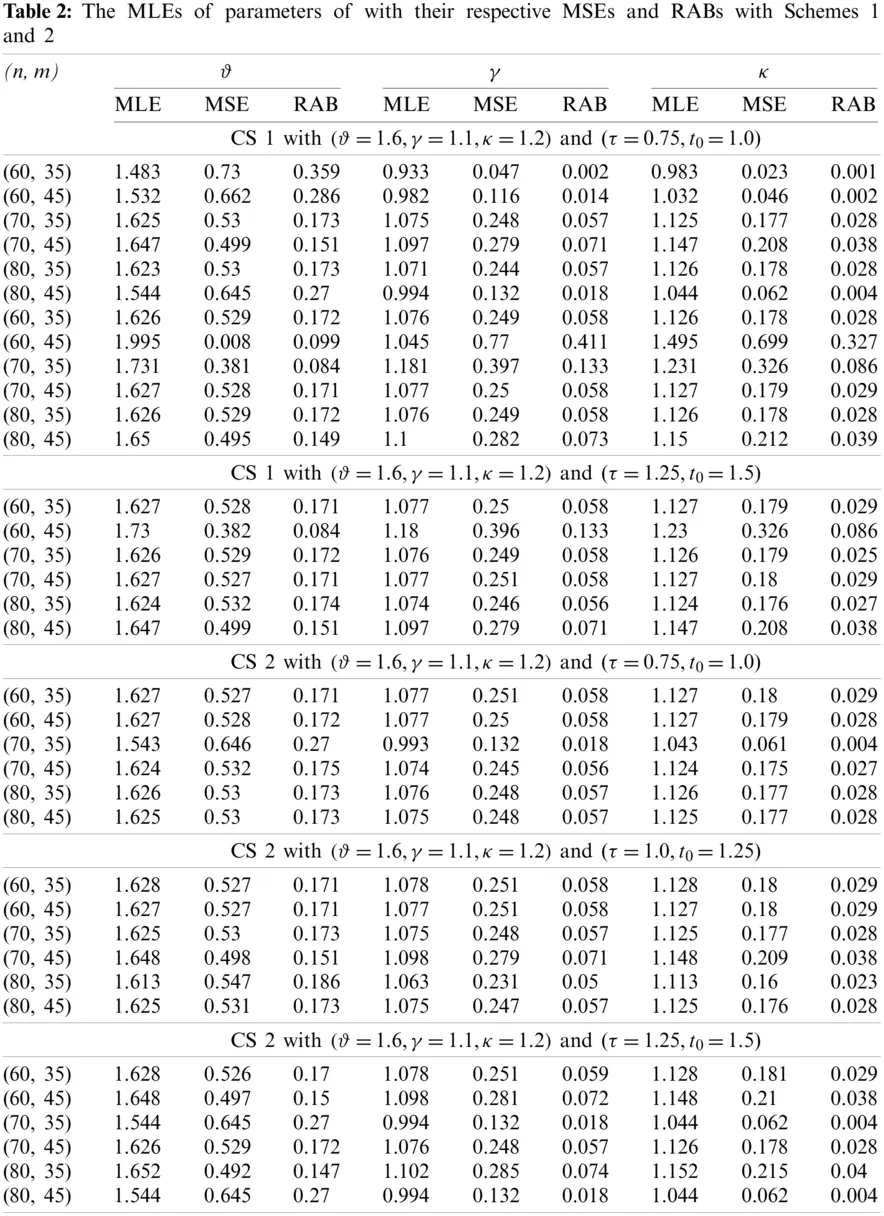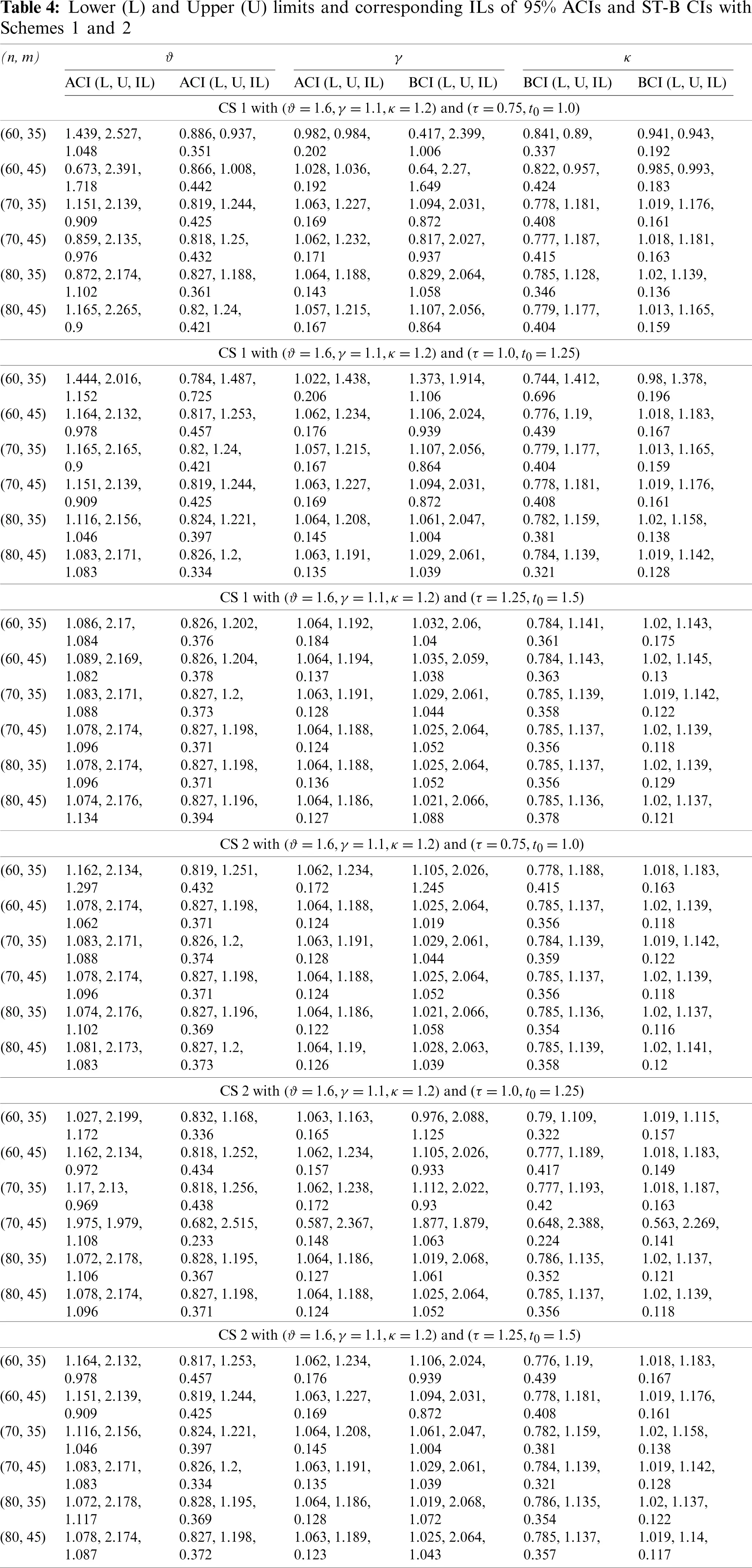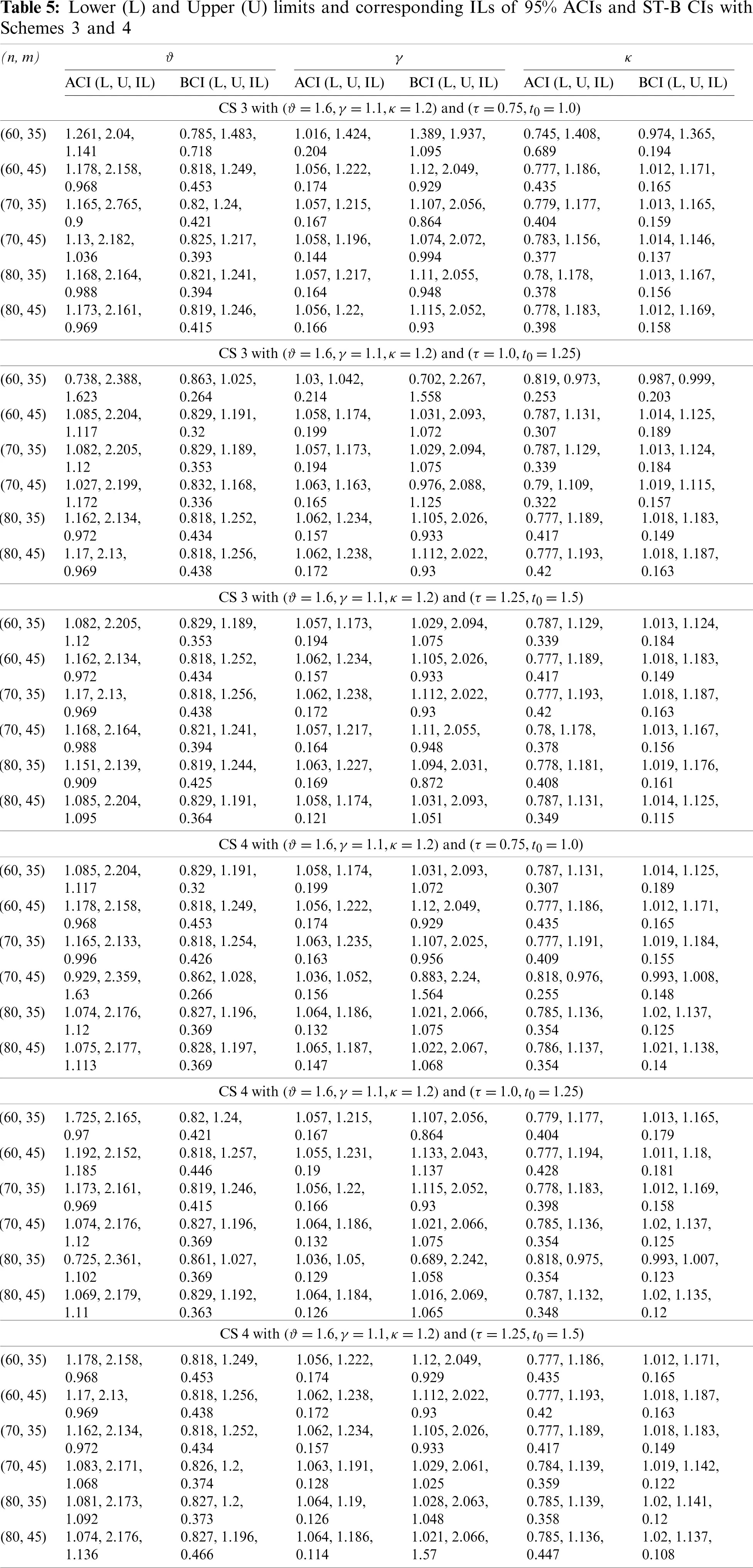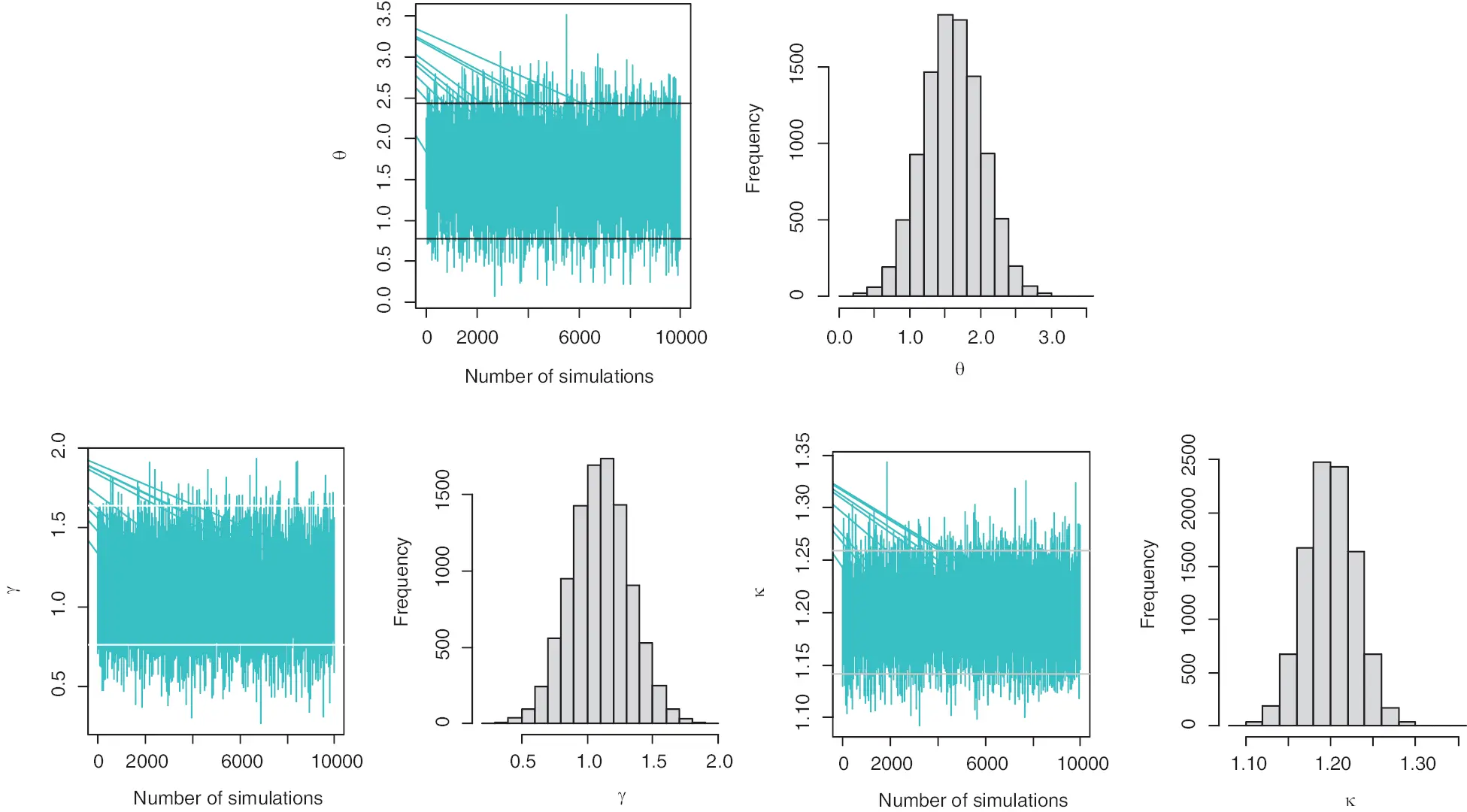Figure 4: The plot of simulated samples and histogram of the parameters (ϑ,γ,κ) respectively with Scheme 1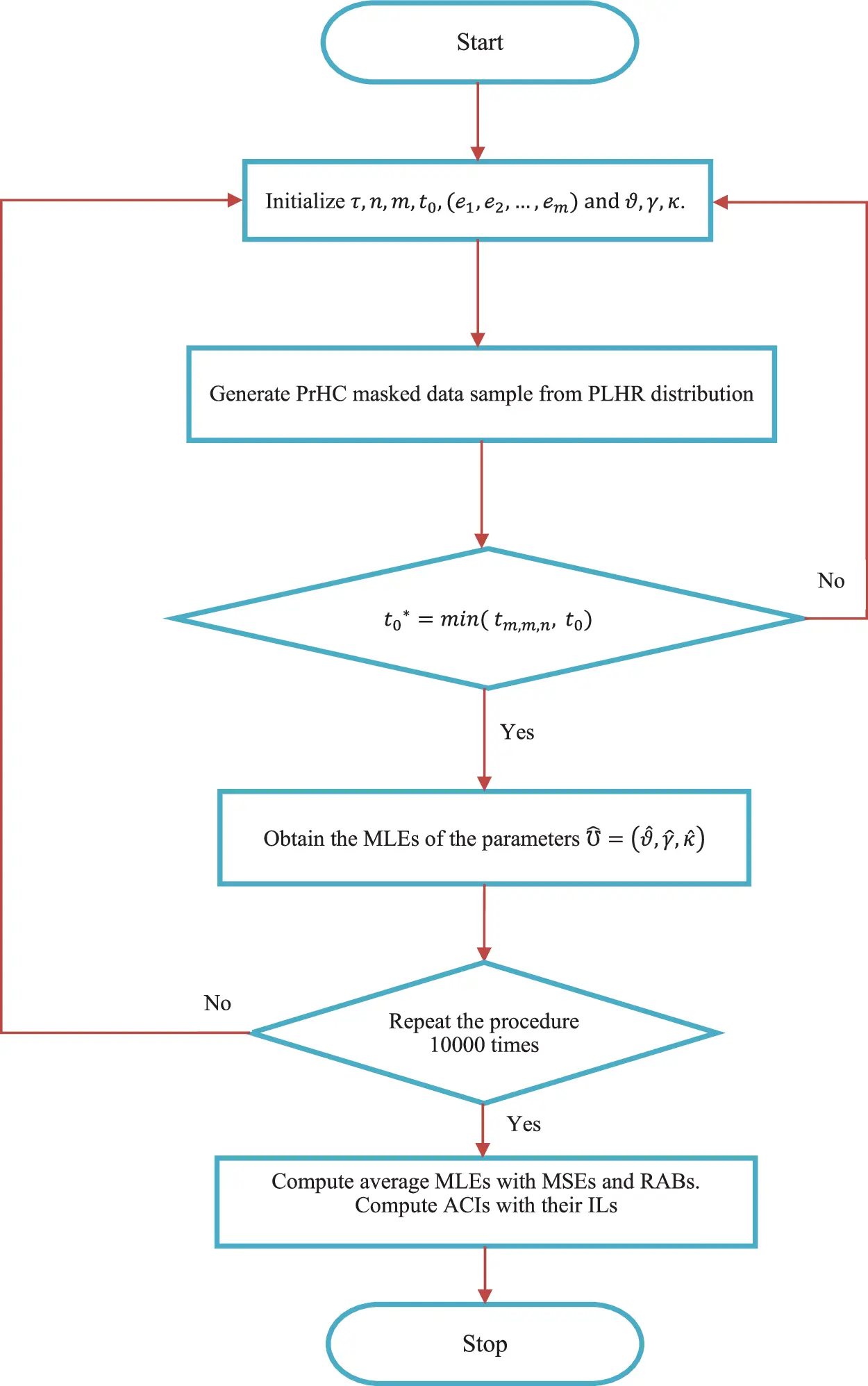Figure 5: The flow chart to demonstrate the proposed estimation method

From the results listed in Tables 2 and 3, it can be observed that, for fixed τ and t0, the MSEs and RABs of the model parameters are decreasing in most of the test schemes as a result of an increase in the values of n and m and MLEs ϑ^,γ^ and κ^ of the model parameters ϑ,γ and κ are getting more closer to their respective real values and this is reasonable because when sample data is large, we can expect more precise estimates. A decreasing pattern in the values of MSEs and RABs can also be observed as n and m increases simultaneously in all cases for fixed values of n,τ and t0. For fixed n and m, the MSEs and RABs are also getting smaller as the values of τ and t0 getting larger and MLEs are approaching to their respective real values and this is also very much possible because with a larger experimental time, we can expect more failures and hence sample size will be larger in that case. Results reported in Tables 4 and 5 shows that expected lengths of both 95% ACIs and ST-B CIs are getting shorter with increasing values of n and m, for fixed values of τ and t0 in almost all cases except for ϑ which is quite acceptable since ϑ is not a parameter of the distribution under study instead it is acceleration factor. It is also observed that both 95% ACIs and ST-B CIs are getting shorter when the values of n and m increases simultaneously. As a comparison between 95% ACIs and ST-B CIs, it is observed that the ST-B CIs provides more narrower expected ILs in almost every case. Therefore, now based on the above findings, we can conclude that the proposed model and methods of estimation in this paper have performed very well, and hence all the statistical assumptions for fitting the model and regarding the estimation are satisfactory.

5  Real Data Application

In this section, an actual data set is utilized to further illustrate the performance of the suggested estimation technique and to demonstrate the application of the PLHR distribution in practice in the field of reliability engineering. The R statistical programming language is used for computation. The dataset reported in Table 6 is an uncensored dataset consisting of real failure times of an airplane’s air conditioning systems (in hours) was first discussed by .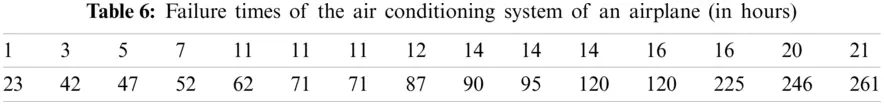The Kolmogorov-Smirnov (K-S) goodness of fit test is used to fit the PLHR distribution to real data. The K-S test is used to compare a given data sample with a reference continuous probability distribution. It is based on the K-S distance, which is the absolute maximum distance between the sample’s empirical distribution function and the reference distribution’s cumulative distribution function and its corresponding p-value. In this current example, the K-S distance was determined to be 0.14146 with a p-value of 0.5856, which is greater than 0.05. Fig. 6 displays the plots of the empirical CDF vs. fitted CDF of PLHR distribution and histogram of data vs. fitted PDF of PLHR distribution. Consequently, it is evident from the K-S distance, p-value and Fig. 6 that the PHLR distribution and considered sample data tabulated in Table 6 both have the same probability distribution. Therefore, given data can be used as an illustration for our model.

Now under SSPALT, for illustrative purposes, let’s consider the stress change time τ to be 24, the test termination time t0 to be 130, and the sample size m to be 20 with respect to the progressive censoring scheme (e1=e2==e6=0, e7 = 1, e8 = 0, e9 = e10 = 1, e11 = 0, e12 = e13 = 1, e14 = 0, e15 = 1, e16 = e17 = 0, e18 = e19 = 0, e20 = 1). So, utilizing the data provided in Table 6 with 0% masking, we have the following PrHC data reported in Table 7 on normal and accelerated stress.

For PrHC data in Table 7 with 0% masking under SSPALT, the MLEs of the parameters with initial values γ=0.042003 and κ=0.3054115 which are the estimates of the parameters based on the complete data. And the initial value of the acceleration factor ϑ is set to be 1.2. The MLEs with their corresponding standard errors are reported in Table 9.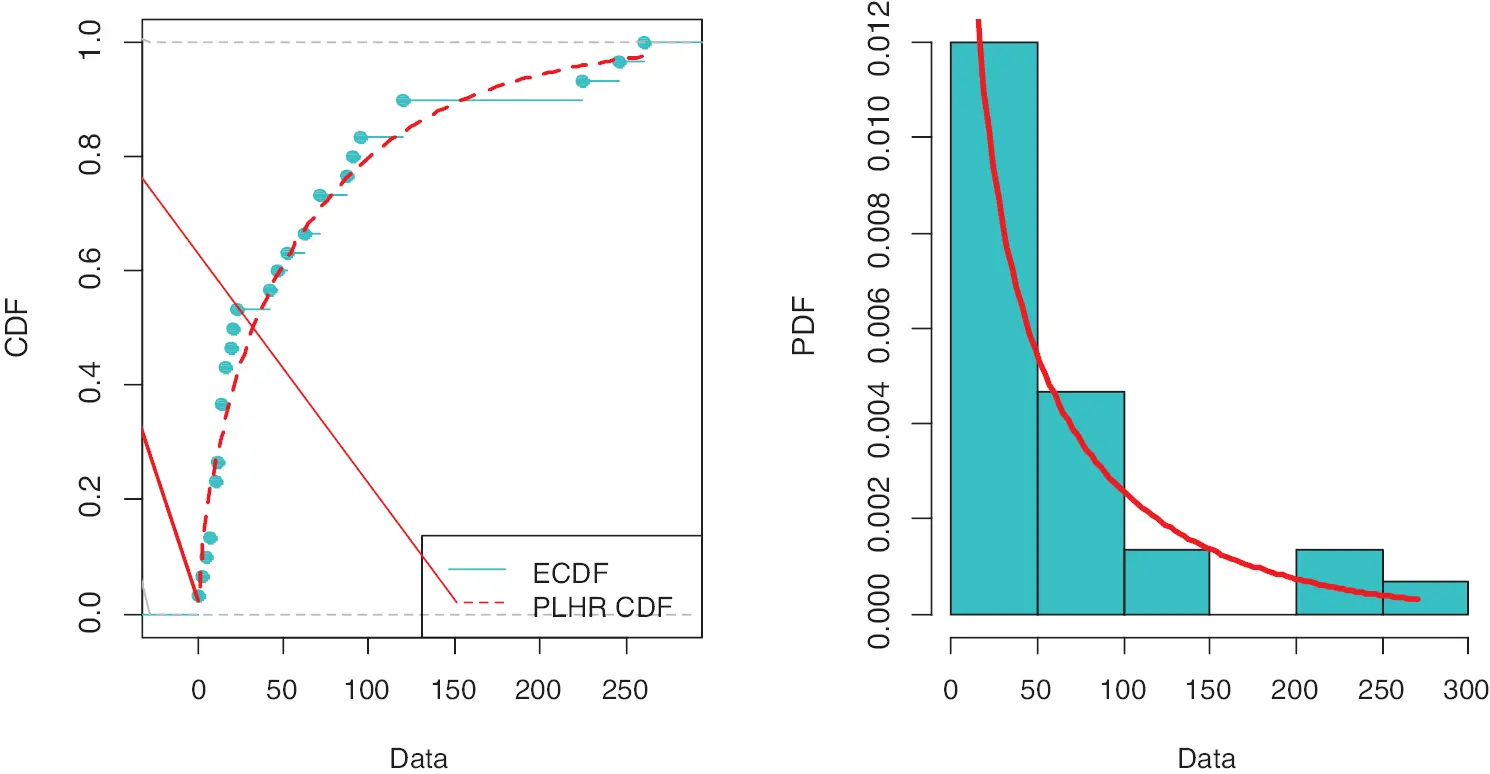Figure 6: Plot of empirical CDF vs. PLHR CDF and histogram of data vs. fitted PDF of the PLHR distribution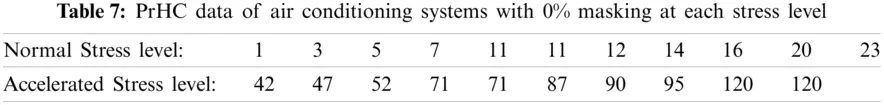Now, to demonstrate the effect of masking, at each stress level, 20% of failed systems are chosen at random to be masked. So, utilizing the data provided in Table 7 with 20% masking, the data obtained after masking with the PrHC scheme under SSPALT at each stress level is reported in the Table 8 as follows: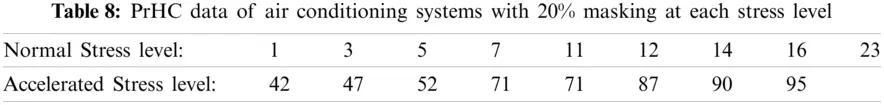For PrHC data in Table 8 with 20% masking under SSPALT, we choose the same initial values of γ,κ and ϑ as it was in the case of 0% masking. The MLEs with their corresponding standard errors are obtained and reported in Table 9.From Table 9, it can be observed that the ML estimates are more accurate with smaller standard errors when the sample size is large or the masking proportion is 0%, than the estimates under a smaller sample size or the masking level is 20%.

6  Conclusions

In this article, the SSPALT model has been developed for PrHC data to analyse the lifetime of a hybrid system of tree components under mask causes of failure. Assuming that the failure of components independently follows PLHR distribution, estimates of the parameters of PLHR distribution and the acceleration factor are then obtained using the MLE technique. The performance of MLEs is investigated through their respective MSEs and RABs. 95% ACIs and ST-B CIs are also constructed and their performance is investigated in terms of their respective ILs. A simulation study has also been conducted to investigate and compare the performance of estimates for the hybrid system under SSPALT for PrHC masked data by utilizing the Monte Carlo simulation technique. Additionally, a real-world data application for an airplane’s air conditioning systems was utilized to demonstrate the proposed approach. As a comparison between 95% ACIs and ST-B CIs, it is observed that the ST-B CIs provide narrower expected ILs in almost every case. Based on the findings, it can be concluded that the proposed model and method of estimation performed well. Hence all the statistical assumptions for fitting the model and regarding the estimation are satisfactory. As a future research project, the present study may be extended to more complex systems using the Bayesian estimation technique with different censored data.

Data Availability: The data used in this paper is available in the paper.

Funding Statement: The authors received no specific funding for this study.

Conflicts of Interest: The authors declare that they have no conflicts of interest to report regarding the present study.

## References

1. Miyakawa, M. (1984). Analysis of incomplete data in competing risks model. IEEE Transactions on Reliability, 33(4), 293-296. [Google Scholar] [CrossRef]
2. Usher, J. S., & Hodgson, T. J. (1988). Maximum likelihood analysis of component reliability using masked system life-test data. IEEE Transactions on Reliability, 37(5), 550-555. [Google Scholar] [CrossRef]
3. Nelson, W. (1990). Accelerated testing: Statistical models, test plans and data analysis. New York: John Wiley & Sons.
4. Ma, H., & Meeker, W. Q. (2010). Strategy for planning accelerated life tests with small sample sizes. IEEE Transactions on Reliability, 59(4), 610-619. [Google Scholar] [CrossRef]
5. Li, X., Chen, W., Sun, F., Liao, H., & Kang, R. (2018). Bayesian accelerated acceptance sampling plans for a lognormal lifetime distribution under Type-I censoring. Reliability Engineering & System Safety, 171(3), 78-86. [Google Scholar] [CrossRef]
6. Han, D., & Bai, T. (2019). On the maximum likelihood estimation for progressively censored lifetimes from constant-stress and step-stress accelerated tests. Electronic Journal of Applied Statistical Analysis, 12(2), 392-404. [Google Scholar] [CrossRef]
7. Bai, X., Shi, Y., Ng, H. K. T. (2020). Statistical inference of Type-I progressively censored step-stress accelerated life test with dependent competing risks. Communications in Statistics-Theory and Methods, 1–27. DOI 10.1080/03610926.2020.1788081. [CrossRef]
8. Kamal, M., Rahman, A., Ansari, S. I., & Zarrin, S. (2020). Statistical analysis and optimum step stress accelerated life test design for Nadarajah Haghighi distribution. Reliability: Theory & Applications, 15(4), 1-9. [Google Scholar] [CrossRef]
9. Rahman, A., Sindhu, T. N., Lone, S. A., & Kamal, M. (2020). Statistical inference for Burr Type X distribution using geometric process in accelerated life testing design for time censored data. Pakistan Journal of Statistics and Operation Research, 16(3), 577-586. [Google Scholar] [CrossRef]
10. Ma, Z., Liao, H., Ji, H., Wang, S., & Yin, F. (2021). Optimal design of hybrid accelerated test based on the inverse Gaussian process model. Reliability Engineering & System Safety, 210(2), 107509. [Google Scholar] [CrossRef]
11. Goel, P. K. (1971). Some estimation problems in the study of tampered random variables (Ph.D. Thesis). Department of Statistics, Carnegie Mellon University, Pittsburgh, Pennsylvania.
12. DeGroot, M. H., & Goel, P. K. (1979). Bayesian estimation and optimal designs in partially accelerated life testing. Naval Research Logistics Quarterly, 26(2), 223-235. [Google Scholar] [CrossRef]
13. Bhattacharyya, G. K., & Soejoeti, Z. (1989). A tampered failure rate model for step-stress accelerated life test. Communications in Statistics-Theory and Methods, 18(5), 1627-1643. [Google Scholar] [CrossRef]
14. Bai, D. S., & Chung, S. W. (1992). Optimal design of partially accelerated life tests for the exponential distribution under Type-I censoring. IEEE Transactions on Reliability, 41(3), 400-406. [Google Scholar] [CrossRef]
15. Bai, D. S., Chung, S. W., & Chun, Y. R. (1993). Optimal design of partially accelerated life tests for the lognormal distribution under Type I censoring. Reliability Engineering & System Safety, 40(1), 85-92. [Google Scholar] [CrossRef]
16. Ismail, A. A. (2012). Inference in the generalized exponential distribution under partially accelerated tests with progressive Type-II censoring. Theoretical and Applied Fracture Mechanics, 59(1), 49-56. [Google Scholar] [CrossRef]
17. Zhang, C., & Shi, Y. (2016). Estimation of the extended Weibull parameters and acceleration factors in the step-stress accelerated life tests under an adaptive progressively hybrid censoring data. Journal of Statistical Computation and Simulation, 86(16), 3303-3314. [Google Scholar] [CrossRef]
18. Ismail, A. A. (2014). Inference for a step-stress partially accelerated life test model with an adaptive Type-II progressively hybrid censored data from Weibull distribution. Journal of Computational and Applied Mathematics, 260(2), 533-542. [Google Scholar] [CrossRef]
19. Nassar, M., Nassr, S. G., & Dey, S. (2017). Analysis of burr Type-XII distribution under step stress partially accelerated life tests with Type-I and adaptive Type-II progressively hybrid censoring schemes. Annals of Data Science, 4(2), 227-248. [Google Scholar] [CrossRef]
20. Mahmoud, M. A., Soliman, A. A., Abd Ellah, A. H., & El-Sagheer, R. M. (2013). Estimation of generalized Pareto under an adaptive Type-II progressive censoring. Intelligent Information Management, 5(3), 73-83. [Google Scholar] [CrossRef]
21. Sobhi, M. M. A., & Soliman, A. A. (2016). Estimation for the exponentiated Weibull model with adaptive Type-II progressive censored schemes. Applied Mathematical Modelling, 40(2), 1180-1192. [Google Scholar] [CrossRef]
22. Hafez, E. H., Riad, F. H., Mubarak, S. A., & Mohamed, M. S. (2020). Study on Lindley distribution accelerated life tests: Application and numerical simulation. Symmetry, 12(12), 2080. [Google Scholar] [CrossRef]
23. Guess, F. M., Usher, J. S., & Hodgson, T. J. (1991). Estimating system and component reliabilities under partial information on cause of failure. Journal of Statistical Planning & Inference, 29(1–2), 75-85. [Google Scholar] [CrossRef]
24. Doganaksoy, N. (1991). Interval estimation from censored and masked system-failure data. IEEE Transactions on Reliability, 40(3), 280-286. [Google Scholar] [CrossRef]
25. Lin, D. K., Usher, J. S., & Guess, F. M. (1993). Exact maximum likelihood estimation using masked system data. IEEE Transactions on Reliability, 42(4), 631-635. [Google Scholar] [CrossRef]
26. Sarhan, A. M. (2001). Reliability estimation of components from masked system life data. Reliability Engineering & System Safety, 74(1), 107-113. [Google Scholar] [CrossRef]
27. Flehinger, B. J., Reiser, B., & Yashchin, E. (2002). Parametric modeling for survival with competing risks and masked failure causes. Lifetime Data Analysis, 8(2), 177-203. [Google Scholar] [CrossRef]
28. Sarhan, A. M. (2003). Estimations of parameters in Pareto reliability model in the presence of masked data. Reliability Engineering & System Safety, 82(1), 75-83. [Google Scholar] [CrossRef]
29. Sarhan, A. M. (2004). Parameter estimations in linear failure rate model using masked data. Applied Mathematics and Computation, 151(1), 233-249. [Google Scholar] [CrossRef]
30. Tan, Z. (2005). Estimation of component failure probability from masked binomial system testing data. Reliability Engineering & System Safety, 88(3), 301-309. [Google Scholar] [CrossRef]
31. Craiu, R. V., & Reiser, B. (2006). Inference for the dependent competing risks model with masked causes of failure. Lifetime Data Analysis, 12(1), 21-33. [Google Scholar] [CrossRef]
32. Tan, Z. (2007). Estimation of exponential component reliability from uncertain life data in series and parallel systems. Reliability Engineering & System Safety, 92(2), 223-230. [Google Scholar] [CrossRef]
33. Liu, Y., & Shi, Y. (2010). Statistical analysis of the reliability for power supply of spacecraft with masked system life test data. Aerospace Control, 28(2), 70-74. [Google Scholar]
34. Reiser, B., Guttman, I., Lin, D. K., Guess, F. M., & Usher, J. S. (1995). Bayesian inference for masked system lifetime data. Journal of the Royal Statistical Society: Series C (Applied Statistics), 44(1), 79-90. [Google Scholar] [CrossRef]
35. Lin, D. K. J., Usher, J. S., & Guess, F. M. (1996). Bayes estimation of component-reliability from masked system-life data. IEEE Transactions on Reliability, 45(2), 233-237. [Google Scholar] [CrossRef]
36. Kuo, L., & Yang, T. Y. (2000). Bayesian reliability modeling for masked system lifetime data. Statistics & Probability Letters, 47(3), 229-241. [Google Scholar] [CrossRef]
37. Sarhan, A. M. (2001). The Bayes procedure in exponential reliability family models using conjugate convex tent prior family. Reliability Engineering & System Safety, 71(1), 97-102. [Google Scholar] [CrossRef]
38. Basu, S., Sen, A., & Banerjee, M. (2003). Bayesian analysis of competing risks with partially masked cause of failure. Journal of the Royal Statistical Society: Series C (Applied Statistics), 52(1), 77-93. [Google Scholar] [CrossRef]
39. Mukhopadhyay, C., & Basu, S. (2007). Bayesian analysis of masked series system lifetime data. Communications in Statistics—Theory and Methods, 36(2), 329-348. [Google Scholar] [CrossRef]
40. Xu, A., & Tang, Y. (2011). Nonparametric Bayesian analysis of competing risks problem with masked data. Communications in Statistics–Theory and Methods, 40(13), 2326-2336. [Google Scholar] [CrossRef]
41. Sarhan, A. M., & Kundu, D. (2008). Bayes estimators for reliability measures in geometric distribution model using masked system life test data. Computational Statistics & Data Analysis, 52(4), 1821-1836. [Google Scholar] [CrossRef]
42. Yousif, Y., Elfaki, F. A., Hrairi, M., & Adegboye, O. A. (2020). A Bayesian approach to competing risks model with masked causes of failure and incomplete failure times. Mathematical Problems in Engineering, 2020, 1-7. [Google Scholar] [CrossRef]
43. Cai, J., Shi, Y., & Liu, B. (2017). Statistical analysis for masked system life data from Marshall-Olkin Weibull distribution under progressive hybrid censoring. Naval Research Logistics, 64(6), 490-501. [Google Scholar] [CrossRef]
44. Peng, W., Huang, H. Z., Xie, M., Yang, Y., & Liu, Y. (2013). A Bayesian approach for system reliability analysis with multilevel pass-fail, lifetime and degradation data sets. IEEE Transactions on Reliability, 62(3), 689-699. [Google Scholar] [CrossRef]
45. Yang, L., Guo, Y., & Wang, Q. (2020). Reliability assessment of a hierarchical system subjected to inconsistent priors and multilevel data. IEEE Transactions on Reliability, 69(1), 277-292. [Google Scholar] [CrossRef]
46. Yang, L., Wang, P., Wang, Q., Bi, S., & Peng, R. (2021). Reliability analysis of a complex system with hybrid structures and multi-level dependent life metrics. Reliability Engineering & System Safety, 209(1–2), 107469. [Google Scholar] [CrossRef]
47. Wang, R., Sha, N., Gu, B., & Xu, X. (2015). Parameter inference in a hybrid system with masked data. IEEE Transactions on Reliability, 64(2), 636-644. [Google Scholar] [CrossRef]
48. Sha, N., Wang, R., Hu, P., & Xu, X. (2015). Statistical inference in dependent component hybrid systems with masked data. Advances in Statistics, 2015, 525136. [Google Scholar] [CrossRef]
49. Cai, J., Shi, Y., & Bai, X. (2017). Statistical analysis of masked data in a hybrid system based on copula theory under progressive hybrid censoring. Sequential Analysis, 36(2), 240-250. [Google Scholar] [CrossRef]
50. Rodrigues, A. S., Pereira, C. A. D. B., & Polpo, A. (2019). Estimation of component reliability in coherent systems with masked data. IEEE Access, 7, 57476-57487. [Google Scholar] [CrossRef]
51. Shi, X., Zhan, P., & Shi, Y. (2020). Statistical inference for a hybrid system model with incomplete observed data under adaptive progressive hybrid censoring. Concurrency and Computation: Practice and Experience, 32(14), e5708. [Google Scholar] [CrossRef]
52. Fan, T. H., & Wang, W. L. (2011). Accelerated life tests for Weibull series systems with masked data. IEEE Transactions on Reliability, 60(3), 557-569. [Google Scholar] [CrossRef]
53. Fan, T. H., & Hsu, T. M. (2012). Accelerated life tests of a series system with masked interval data under exponential lifetime distributions. IEEE Transactions on Reliability, 61(3), 798-808. [Google Scholar] [CrossRef]
54. Xu, A., Basu, S., & Tang, Y. (2014). A full Bayesian approach for masked data in step-stress accelerated life testing. IEEE Transactions on Reliability, 63(3), 798-806. [Google Scholar] [CrossRef]
55. Xu, A., Tang, Y., & Guan, Q. (2014). Bayesian analysis of masked data in step-stress accelerated life testing. Communications in Statistics-Simulation and Computation, 43(8), 2016-2030. [Google Scholar] [CrossRef]
56. Shi, X., Liu, Y., & Shi, Y. (2017). Statistical analysis for masked hybrid system lifetime data in step-stress partially accelerated life test with progressive hybrid censoring. PLOS One, 12(10), e0186417. [Google Scholar] [CrossRef]
57. Shi, X., Shi, Y., & Song, Q. (2018). Inference for four-unit hybrid system with masked data under partially acceleration life test. Systems Science & Control Engineering, 6(1), 195-206. [Google Scholar] [CrossRef]
58. Shi, X. L., Lu, P., & Shi, Y. M. (2018). Inference and optimal design on step-stress partially accelerated life test for hybrid system with masked data. Journal of Systems Engineering and Electronics, 29(5), 1089-1100. [Google Scholar] [CrossRef]
59. Rinne, H. (2014). The hazard rate: Theory and inference (with supplementary MATLAB-programs). Justus-Liebig-University, Germany. http://geb.uni-giessen.de/geb/volltexte/2014/10793/.
60. Tarvirdizade, B., & Nematollahi, N. (2019). The power-linear hazard rate distribution and estimation of its parameters under progressively Type-II censoring. Hacettepe Journal of Mathematics and Statistics, 48(3), 818-844. [Google Scholar] [CrossRef]
61. Kundu, D., & Joarder, A. (2006). Analysis of Type-II progressively hybrid censored data. Computational Statistics & Data Analysis, 50(10), 2509-2528. [Google Scholar] [CrossRef]
62. Balakrishnan, N. (2007). Progressive censoring methodology: An appraisal. Test, 16(2), 211-296. [Google Scholar] [CrossRef]
63. Balakrishnan, N., & Kundu, D. (2013). Hybrid censoring: Models, inferential results and applications. Computational Statistics & Data Analysis, 57(1), 166-209. [Google Scholar] [CrossRef]
64. Efron, B. (1982). The jackknife, the bootstrap and other resampling plans. Philadelphia, PA, USA: Society for Industrial and Applied Mathematics.
65. Hall, P. (1988). Theoretical comparison of bootstrap confidence intervals. The Annals of Statistics, 16(3), 927-953. [Google Scholar] [CrossRef]
66. Efron, B., Tibshirani, R. J. (1993). An introduction to the bootstrap (1st ed.). London, UK: Chapman and Hall/CRC. DOI 10.1201/9780429246593. [CrossRef]
67. Balakrishnan, N., & Sandhu, R. A. (1995). A simple simulation algorithm for generating progressive Type-II censored samples. The American Statistician, 49(2), 229-230. [Google Scholar] [CrossRef]
68. Linhart, H., Zucchini, W. (1986). Model selection. New York: Wiley.

Appendix A

Elements of F are:

2ϑ2=r(rnu)(t0τ)2(1+κγΨ0κ1)(t0τ){2r(rnu)(t0τ)(Ψ0+γΨ0κ)(1ϕ0)ϕ0(1(1ϕ0)2)}×{(1+κγΨ0κ1)(Ψ0+γΨ0κ)+(Ψ0+γΨ0κ)ϕ0(1ϕ0)(Ψ0+γΨ0κ)+2(Ψ0+γΨ0κ)(1ϕ0)ϕ0(1(1ϕ0)2)}(rnu)ϑ2+i=nu+1r(1+ri)(tiτ)2(1+κγΨ2κ1)+i=nu+1r(tiτ){(1+κγΨ2κ1)(Ψ2+γΨ2κ)}×{γκ(κ1)Ψ2κ2(1+κγΨ2κ1)(1+κγΨ2κ1)(Ψ2+γΨ2κ)}i=nu+1r(tiτ){2(1+ri)(Ψ2+γΨ2κ)(1ϕ1)ϕ1(1(1ϕ1)2)}×{(1+κγΨ2κ1)(Ψ2+γΨ2κ)+ϕ1(Ψ2+γΨ2κ)(1ϕ1)(Ψ2+γΨ2κ)+2(Ψ2+γΨ2κ)(1ϕ1)ϕ1(1(1ϕ1)2)}2γ2=ϕ0Ψ0κ+1(κ+1)(1ϕ0)Ψ0κ+1(κ+1)+2(1ϕ0)ϕ0Ψ0κ+1(κ+1)(1(1ϕ0)2)i=1nu{tiκ(ti+γtiκ)}2i=nu+1r{Ψ2κΨ2+γΨ2κ}2+i=1nu{tiκ+1Ψ1(κ+1){1Ψ1}tiκ+1(κ+1)+2tiκ+1(1Ψ1)Ψ1(κ+1){1(1Ψ1)2}}+i=nu+1r{Ψ2κ+1ϕ1(κ+1){1ϕ1}Ψ2κ+1(κ+1)+2Ψ2κ+1(1ϕ1)ϕ1(κ+1){1(1ϕ1)2}} 2κ2=r*(r-nu)γ{Ψ0κ+1Λ0(κ+1)2}{logΨ0-logΨ0Λ0-2(κ+1)}-{r*(r-nu)γ2Ψ0κ+1Λ0(1-ϕ0)(κ+1)2(1-(1-ϕ0)2)}×{logΨ0-logΨ0Λ0-Ψ0κ+1ϕ0γΛ0(κ+1)2(1-ϕ0)-2(κ+1)-2Ψ0κ+1(1-ϕ0)ϕ0γΛ0(κ+1)2(1-(1-ϕ0)2)}+i=1nuγtiκlogti(ti+γtiκ){logti-γtiκlogti(ti+γtiκ)}+i=1nu(1+ri)γ{tiκ+1Λ1(κ+1)2}{logti-logtiΛ1-2(κ+1)}-i=1nu(1+ri)γ2tiκ+1Λ1(1-Ψ1)(κ+1)2(1-(1-Ψ1)2)×{logti-logtiΛ1-γtiκ+1Λ1Ψ1(κ+1)2(1-Ψ1)-2(κ+1)-2γtiκ+1Λ1(1-Ψ1)Ψ1(κ+1)2(1-(1-Ψ1)2)}+i=nu+1rγΨ2κ+1logΨ2(Ψ2+γΨ2κ){logΨ2-γΨ2κlogΨ2(Ψ2+γΨ2κ)}+i=nu+1r(1+ri)γ{Ψ2κ+1Λ2(κ+1)2}×{logΨ2-logΨ2Λ2-2(κ+1)}-i=nu+1r(1+ri)γ2Ψ2κ+1Λ2(1-ϕ1)(κ+1)2(1-(1-ϕ1)2)×{logΨ2-logΨ2Λ2-γΨ2κ+1Λ2ϕ1(κ+1)2(1-ϕ1)-2(κ+1)-2γΨ2κ+1Λ2(1-ϕ1)ϕ1(κ+1)2(1-(1-ϕ1)2)}2ϑγ=2γϑ=-r*(r-nu)(t0-τ)Ψ0κ-i=nu+1r(1+ri)(ti-τ)Ψ2κ+{2r*(r-nu)(t0-τ)Ψ0κ+1(1-ϕ0)ϕ0(κ+1)(1-(1-ϕ0)2)((κ+1)Ψ0+(Ψ0+γΨ0κ)ϕ0(1-ϕ0)+2(Ψ0+γΨ0κ)(1-ϕ0)ϕ0(1-(1-ϕ0)2))}+i=nu+1r{Ψ2κ(ti-τ)(Ψ2+γΨ2κ)(κΨ2-(1+γκΨ2κ-1)(Ψ2+γΨ2κ))}+i=nu+1r{2(1+ri)(ti-τ)Ψ2κ+1(1-ϕ1)ϕ1(κ+1)(1-(1-ϕ1)2)×((κ+1)Ψ2+(Ψ2+γΨ2κ)ϕ1(1-ϕ1)+2(Ψ2+γΨ2κ)(1-ϕ1)ϕ1(1-(1-ϕ1)2))}2ϑκ=2κϑ=r*(r-nu)γ{(κ+1)(t0-τ)Λ0Ψ0κ(κ+1)2}{1-1logΛ0}-r*(r-nu)γ{2(t0-τ)Ψ0κ+1Λ0(1-ϕ0)(κ+1)2(1-(1-ϕ0)2)}{(κ+1)Ψ0(1-1logΛ0)+((Ψ0+γΨ0κ)ϕ0(1-ϕ0))+(2(Ψ0+γΨ0κ)(1-ϕ0)ϕ0(1-(1-ϕ0)2))}+i=nu+1r{γ(ti-τ)Ψ2κlogΨ2Ψ2(Ψ2+γΨ2κ)(κ+1logΨ2-(1+κγΨ2κ)(Ψ2+γΨ2κ))}+i=nu+1r{(1+ri)(ti-τ)γΨ2κ+1Λ2(κ+1)Ψ2(1-1logΛ2)}-i=nu+1r{2(1+ri)(ti-τ)γΨ2κ+1Λ2(1-ϕ1)(κ+1)2(1-(1-ϕ1)2)((κ+1)Ψ2-(κ+1)Ψ2logΛ2+(Ψ2+γΨ2κ)ϕ1(1-ϕ1)+2(Ψ2+γΨ2κ)(1-ϕ1)ϕ1(1-(1-ϕ1)2))}2γκ=2κγ={r*(r-nu)Ψ0κ+1Λ0(κ+1)2}-{2r*(r-nu)γΨ0κ+1Λ0(1-ϕ0)(κ+1)2(1-(1-ϕ0)2)(1γ+Ψ0κ+1ϕ0(κ+1)(1-ϕ0)+2Ψ0κ+1(1-ϕ0)ϕ0(κ+1){1-(1-ϕ0)2})}+i=1nu{γtiκlogti(ti+γtiκ)(1γ-tiκ(ti+γtiκ))}+i=1nu{(1+ri)tiκ+1Λ1(κ+1)2}-i=1nu{2γ(1+ri)tiκ+1Λ1(1-Ψ0)(κ+1)2(1-(1-Ψ0)2)(1γ+Ψ0tiκ+1(κ+1)(1-Ψ0)+2{1-Ψ0}Ψ0tiκ+1(κ+1)(1-(1-Ψ0)2))}+i=nu+1r{γΨ2κlogΨ2(Ψ2+γΨ2κ)(1γ-Ψ2κ(Ψ2+γΨ2κ))}+i=nu+1r{(1+ri)Ψ2κ+1Λ2(κ+1)2}-i=nu+1r{2(1+ri)γΨ2κ+1Λ2(1-ϕ1)(κ+1)2(1-(1-ϕ1)2)(1γ+Ψ2κ+1ϕ1(κ+1)(1-ϕ1)+2Ψ2κ+1(1-ϕ1)ϕ1(κ+1)(1-(1-ϕ1)2))}

where, (1(κ+1)logΨ0)=Λ0; (1(κ+1)logti)=Λ1; (1(κ+1)logΨ2)=Λ2.This work is licensed under a Creative Commons Attribution 4.0 International License, which permits unrestricted use, distribution, and reproduction in any medium, provided the original work is properly cited.